# How to Calculate the Upper and lower control limits in Microsoft Excel

In this tutorial, we will learn how to calculate the upper and lower limits in Microsoft Excel. To calculate the upper and lower control boundaries the AVERAGE and ST.DEV functions are commonly used.

Let’s use a sample dataset of process measurements to demonstrate how to calculate lower and upper control limits in Excel.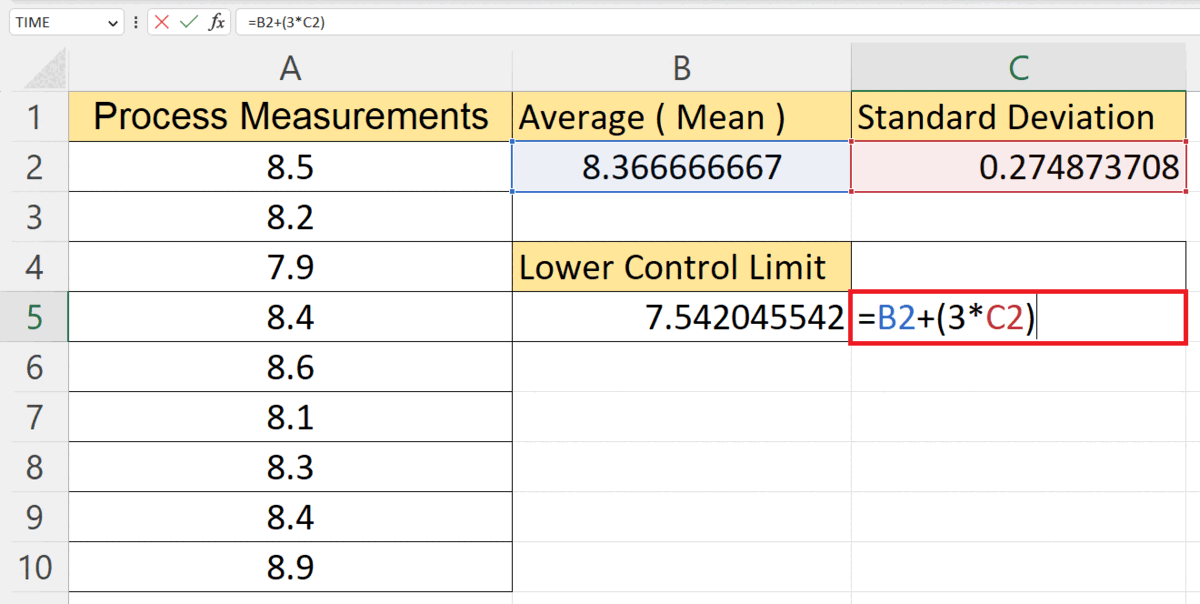Upper and lower control limits in Excel are statistical boundaries used in process control charts to assess if a process is within an acceptable range or exhibiting special causes of variation. They help identify deviations from desired performance based on process data, enabling users to monitor processes and take necessary actions for optimal performance.

### Step 1 – Calculate the Mean or the Average of the Data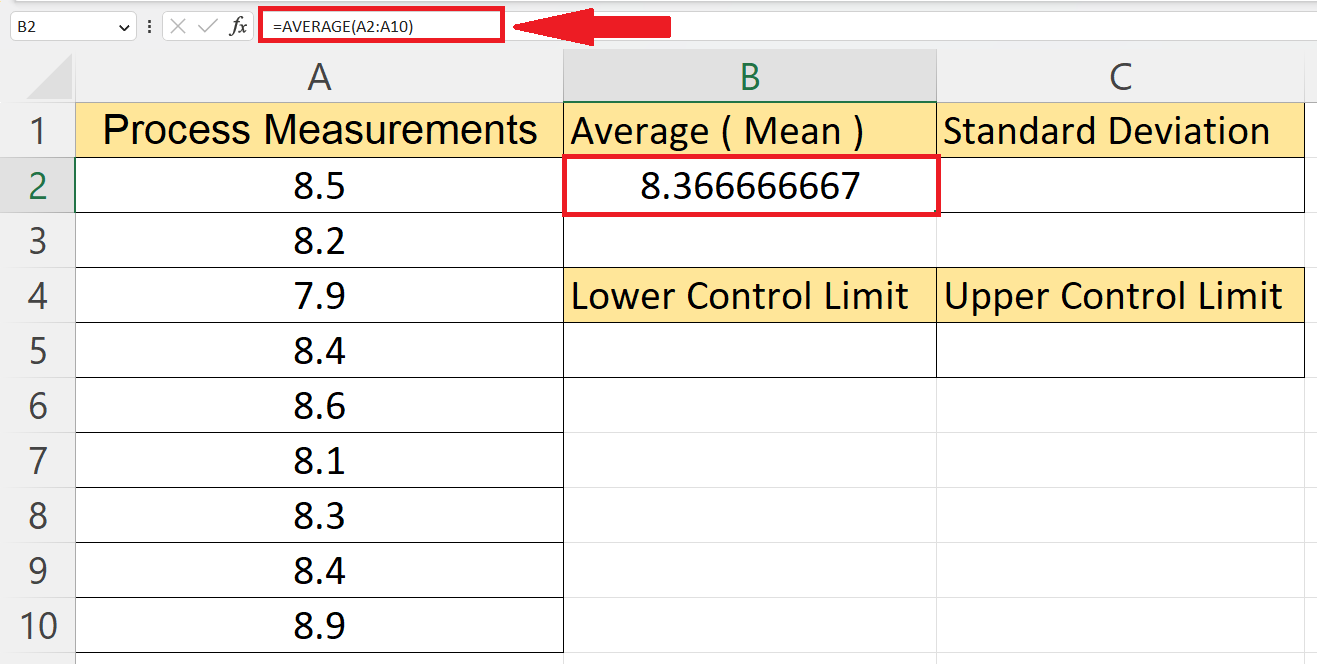– Calculate the mean or the average of the data utilizing the AVERAGE function.
– The structure of the AVERAGE function is:
AVERAGE(A2:A10)
– Where the range A2:A10 contains the data.
– Hit the Enter key to calculate the average.

### Step 2 – Choose Another Cell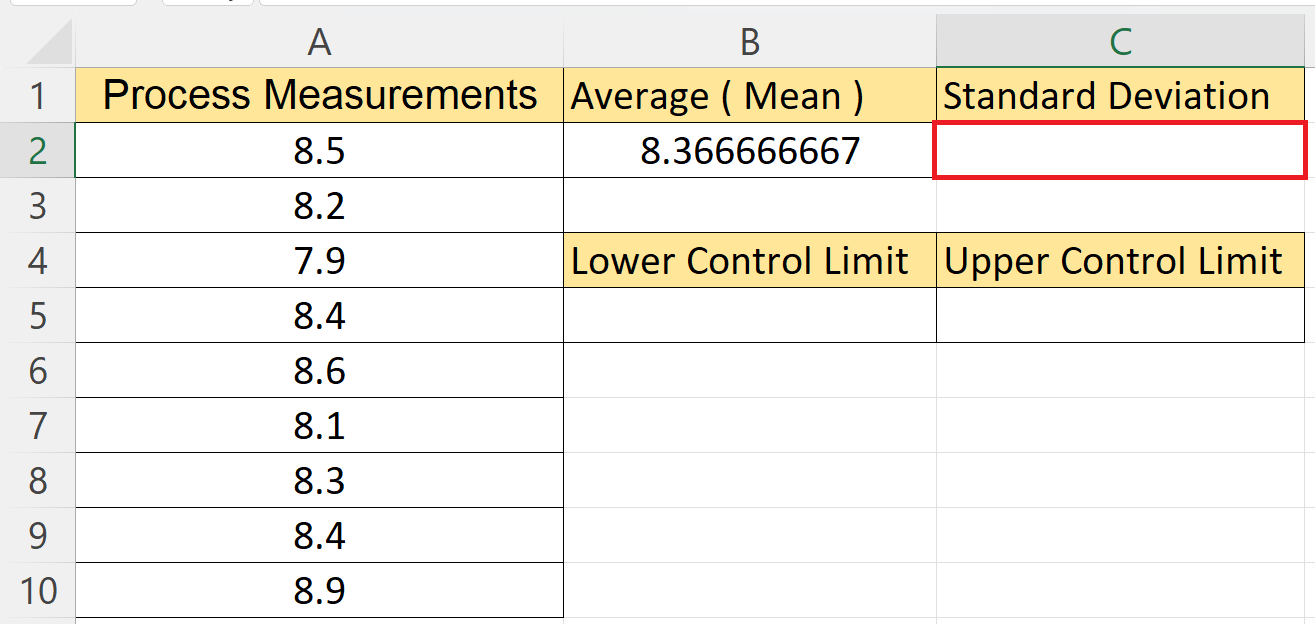– Choose another cell to calculate the standard deviation.

### Step 3 – Utilize the STDEV.P Function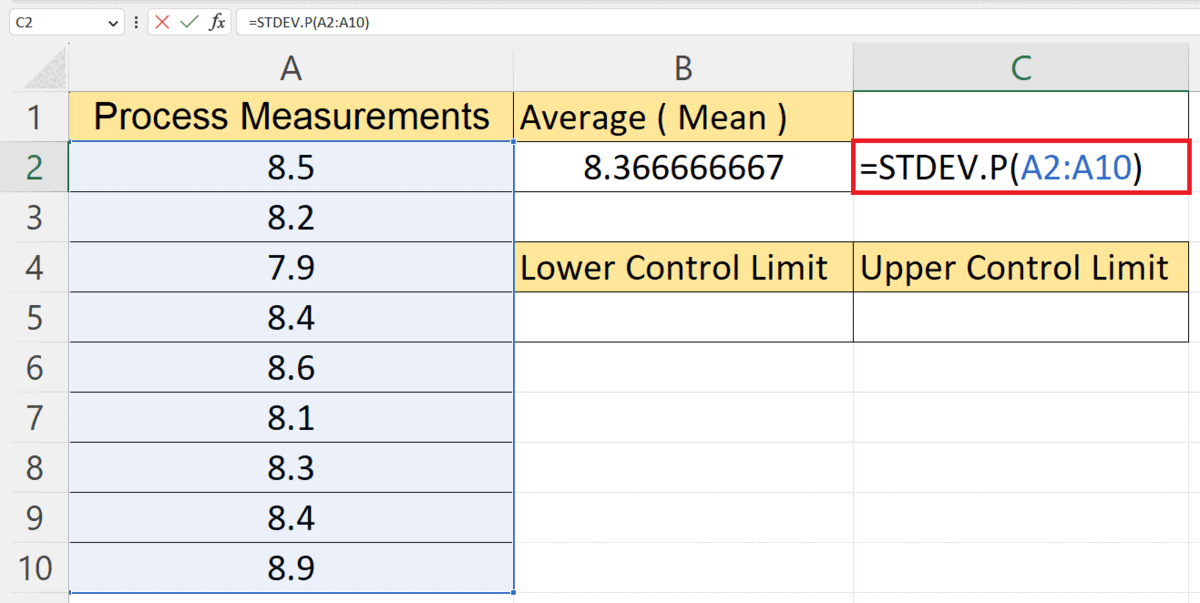– Utilize the STDEV.P function to calculate the standard deviation.
STRDEV.P(A2:A10)
– The range A2:A10 contains the data.

### Step 4 – Choose a Targeted Cell to Calculate the Lower Control Limit– Choose a blank targeted cell.

### Step 5 – Calculate the Lower Control Limit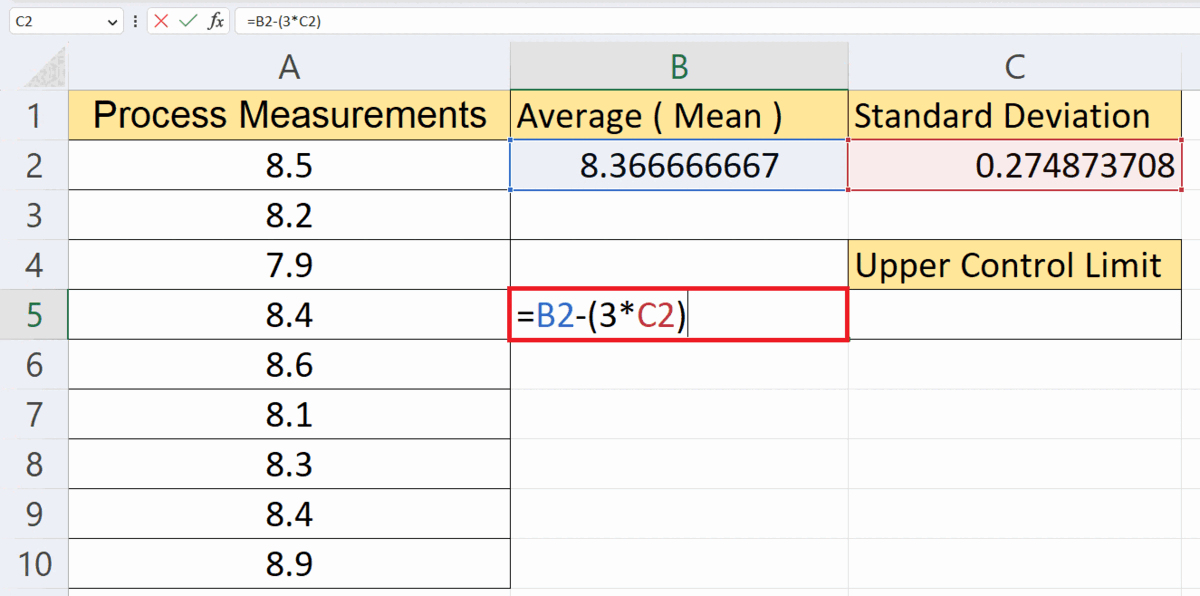– Calculate the lower control limit utilizing the formula:
B2 – (3*C2)
– Where the cells B2 and C2 contain the average and the standard deviation respectively.
– Parameter 3 is the number of standard deviations to be used.
– Hit the Enter key.

### Step 6 – Choose a Targeted Cell to Calculate the Upper Control Limit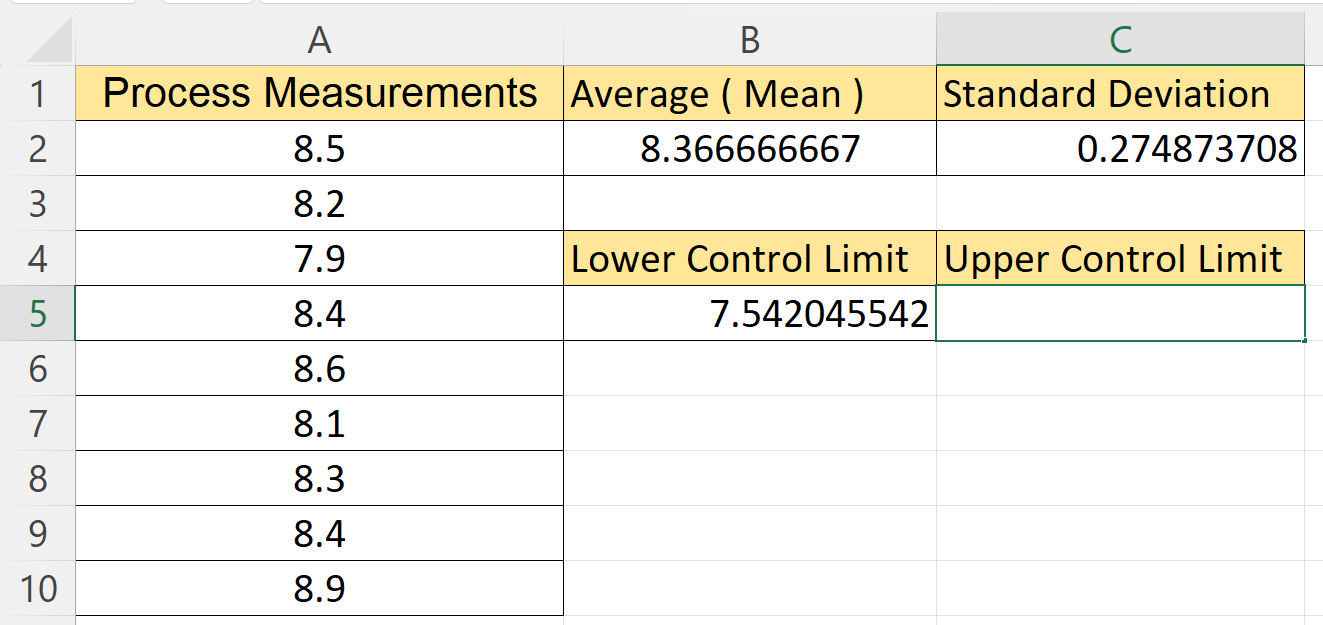– Choose a blank targeted cell.

### Step 7 – Calculate the Lower Control Limit– Calculate the lower control limit utilizing the formula:
B2 + (3*C2)
– Where the cells B2 and C2 contain the average and the standard deviation respectively.
– Parameter 3 is the number of standard deviations to be used.
– Hit the Enter key.